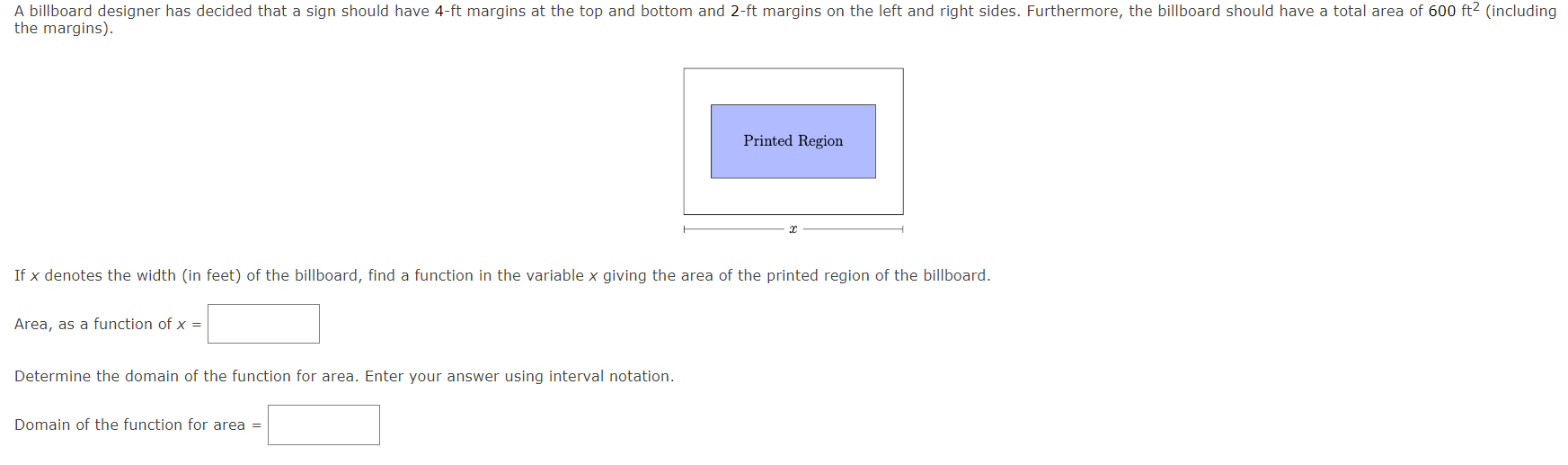Home / Expert Answers / Calculus / if-x-denotes-the-width-in-feet-of-the-billboard-find-a-function-in-the-variable-x-g-pa615

# (Solved): If \( x \) denotes the width (in feet) of the billboard, find a function in the variable \( x \) g ...If \( x \) denotes the width (in feet) of the billboard, find a function in the variable \( x \) giving the area of the printed region of the billboard. Area, as a function of \( x= \) Determine the domain of the function for area. Enter your answer using interval notation. Domain of the function for area \( = \)

We have an Answer from Expert